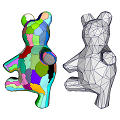CGAL 4.14 - Triangulated Surface Mesh Approximation
Triangulated Surface Mesh Approximation ReferencePierre Alliez, David Cohen-Steiner, Lingjie Zhu
This package implements the Variational Shape Approximation method to approximate an input surface triangle mesh by a simpler surface triangle mesh. The algorithm proceeds by iterative clustering of triangles, the clustering process being seeded randomly, incrementally or hierarchically. While the default function runs an automated version of the algorithm, interactive control is possible via a class interface. The API is flexible and can be extended to user-defined proxies and error metrics.
Introduced in: CGAL 4.14
BibTeX: cgal:az-tsma-19a
Depends on: Eigen
Windows Demo: Polyhedron demo
Common Demo Dlls: dlls

## Parameters

Optional parameters of the functions of this package are implemented as BGL named parameters. The page Named Parameters describes their usage and provides the list of parameters used in this package.

## Concepts

• ErrorMetricProxy

## Main Functions

• CGAL::Surface_mesh_approximation::approximate_triangle_mesh()

## Classes

• CGAL::Surface_mesh_approximation::L21_metric_plane_proxy
• CGAL::Surface_mesh_approximation::L2_metric_plane_proxy
• CGAL::Variational_shape_approximation

## Modules

Named Parameters
How to use BGL Optional Named Parameters

Concepts

## Classes

class  CGAL::Surface_mesh_approximation::L21_metric_plane_proxy< TriangleMesh, VertexPointMap, GeomTraits >
Approximation L21 metric of vector proxy. More...

class  CGAL::Surface_mesh_approximation::L2_metric_plane_proxy< TriangleMesh, VertexPointMap, GeomTraits >
Approximation L2 metric of plane proxy. More...

class  CGAL::Variational_shape_approximation< TriangleMesh, VertexPointMap, ErrorMetricProxy, GeomTraits, Concurrency_tag >
Main class for Variational Shape Approximation algorithm. More...

## Enumerations

enum  CGAL::Surface_mesh_approximation::Verbose_level { CGAL::Surface_mesh_approximation::SILENT, CGAL::Surface_mesh_approximation::MAIN_STEPS, CGAL::Surface_mesh_approximation::VERBOSE }
Verbose level enumeration. More...

enum  CGAL::Surface_mesh_approximation::Seeding_method { CGAL::Surface_mesh_approximation::RANDOM, CGAL::Surface_mesh_approximation::INCREMENTAL, CGAL::Surface_mesh_approximation::HIERARCHICAL }
Seeding method enumeration for Variational Shape Approximation algorithm. More...

## Functions

template<typename TriangleMesh , typename NamedParameters >
bool CGAL::Surface_mesh_approximation::approximate_triangle_mesh (const TriangleMesh &tm, const NamedParameters &np)
approximates the input mesh with plane proxies. More...

## ◆ Seeding_method

#include <CGAL/Variational_shape_approximation.h>

Seeding method enumeration for Variational Shape Approximation algorithm.

Enumerator
RANDOM

Random seeding.

INCREMENTAL

Incremental seeding.

HIERARCHICAL

Hierarchical seeding.

## ◆ Verbose_level

#include <CGAL/Surface_mesh_approximation/approximate_triangle_mesh.h>

Verbose level enumeration.

Enumerator
SILENT

Silent.

MAIN_STEPS

Main steps.

VERBOSE

Verbose.

## ◆ approximate_triangle_mesh()

template<typename TriangleMesh , typename NamedParameters >
 bool CGAL::Surface_mesh_approximation::approximate_triangle_mesh ( const TriangleMesh & tm, const NamedParameters & np )

#include <CGAL/Surface_mesh_approximation/approximate_triangle_mesh.h>

approximates the input mesh with plane proxies.

This function uses the Variational Shape Approximation algorithm described in  to approximate a triangle surface mesh, with indexed triangles as output.

Template Parameters
 TriangleMesh model of FaceListGraph NamedParameters a sequence of Named Parameters
Parameters
 tm triangle surface mesh to be approximated np optional sequence of Named Parameters among the ones listed below
Returns
true if the indexed triangles represent a 2-manifold, oriented surface mesh, and false otherwise.
Approximation Named Parameters
 geom_traits a geometric traits class instance, model of Kernel. Exact constructions kernels are not supported by this function. vertex_point_map the property map with the points associated to the vertices of tm. Instance of a class model of ReadablePropertyMap. verbose_level set verbose level. seeding_method selection of seeding method. max_number_of_proxies maximum number of proxies to approximate the input mesh. min_error_drop minimum error drop of the approximation, expressed in ratio between two iterations of proxy addition. number_of_relaxations number of relaxation iterations interleaved within seeding. number_of_iterations number of partitioning and fitting iterations after seeding.
Meshing Named Parameters
 subdivision_ratio chord subdivision ratio threshold to the chord length or average edge length. relative_to_chord if true the subdivision_ratio is the ratio of the furthest vertex distance to the chord length, otherwise is the average edge length. with_dihedral_angle if set to true the subdivision_ratio is weighted by dihedral angle. optimize_anchor_location if set to true, optimize the anchor locations. pca_plane set true if use PCA plane fitting, otherwise use the default area averaged plane parameters.
Output Named Parameters
 face_proxy_map a WritablePropertyMap with boost::graph_traits::face_descriptor as key and std::size_t as value type. A proxy is a set of connected faces which are placed under the same proxy patch (see Figure 71.3). The proxy-ids are contiguous in range [0, number_of_proxies - 1]. proxies output iterator over proxies. anchors output iterator over anchor points. triangles output iterator over indexed triangles.
Examples:
Surface_mesh_approximation/vsa_approximation_2_example.cpp, Surface_mesh_approximation/vsa_approximation_example.cpp, Surface_mesh_approximation/vsa_segmentation_example.cpp, and Surface_mesh_approximation/vsa_simple_approximation_example.cpp.